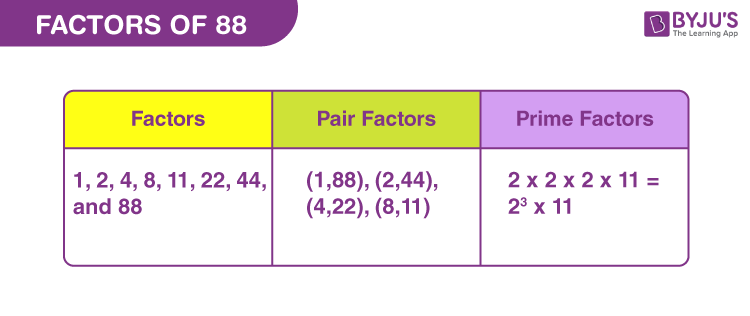# Factors of 88

In Maths, factors of 88 are the natural numbers that result in zero remainder. It means the factor divides the original number, uniformly. Thus, they are also called divisors of 88. Also, when the multiplication on two such numbers is equal to 88, then the numbers are called factors.

88 is a double digit number, that is the same if read forward and backward. Such numbers are also called palindrome. 88 is also an even composite number that has more than two factors. It means 88 is divisible by more than two natural numbers.

We can easily find the factors of 88 by the division method and prime factorisation method. Let us find the factors in pairs of 88 and the prime factors.## How to Find the Factors of 88?

The factors of 88 split or break the number into equal parts. Thus, we need to divide 88 by such numbers, which results in zero remainder.

88 ÷ 1 = 88 [Remainder = 0]

88 ÷ 2 = 41 [Remainder = 0]

88 ÷ 4 = 22 [Remainder = 0]

88 ÷ 8 = 11 [Remainder = 0]

88 ÷ 11 = 8 [Remainder = 0]

88 ÷ 22 = 4 [Remainder = 0]

88 ÷ 44 = 2 [Remainder = 0]

88 ÷ 88 = 1 [Remainder = 0]

Thus, the factors of 88 are 1, 2, 4, 8, 11, 22, 44, and 88.

## Pair Factors of 88

The pair factors of 88 are the integers that result in 88, when multiplied together. Therefore, such pairs of factors are:

1 × 88 = 88

2 × 44 = 88

4 × 22 = 88

8 × 11 = 88

Therefore, the pair factors are (1,88), (2,44), (4,22), (8,11).

The pair factors could be considered as negative integers also, since we can get a positive value after multiplying the two negative numbers.

-1 × -88 = 88

-2 × -44 = 88

-4 × -22 = 88

-8 × -11 = 88

Therefore, the negative pair factors of 88 are (-1,-88), (-2,-44), (-4,-22), (-8,-11).

## Prime Factorisation of 88

Prime factorisation of 88 results in the prime factors of the original number. These prime factors are the prime numbers that can easily divide the original number. Let us list down the prime numbers from 1 to 88, to find the prime factors.

 88 is divisible 2 88 ÷ 2 = 44 44 is again divisible by 2 44 ÷ 2 = 22 22 is an even number and divisible by 2 22 ÷ 2 = 11 Now, 11 is a prime number and is divisible by itself 11 ÷ 11 = 1

Therefore, the prime factors of 88 are 2 and 11.

 Prime factorisation of 88 = 2 × 2 × 2 × 11 Exponential Form = 23 × 11

## Solved Examples

Q.1: Find the sum of all the factors of 88.

Solution: The factors of 88 are 1, 2, 4, 8, 11, 22, 44, and 88 .

Sum = 1 + 2 + 4 + 8 + 11 + 22 + 44 + 88 = 180

Therefore, 180 is the required sum.

Q.2: If there are 88 balls to be divided into four equal parts., how many balls does each part have?

Solution: Given,

Number of balls = 88

We need to divide 88 balls into 4 equal parts

Number of balls, each part will have = 88/4 = 22

Q.3: What is the greatest common factor of 88 and 121?

Answer: Let us first write the factors of both the numbers.

88 → 1, 2, 4, 8, 11, 22, 44, 88

121 → 1, 11, 121

GCF (88, 121) = 11

Q.4: What are the common factors of 88 and 120?

Answer: The factors of both the numbers are:

88 →1, 2, 4, 8, 11, 22, 44, 88

120 → 1, 2, 3, 4, 5, 6, 8, 10, 12, 15, 20, 24, 30, 40, 60, 120

Therefore, by comparing we get the common factors as: 1, 2, 4 and 8.

## Practice Questions

1. What are the even divisors of 88?
2. What is the GCF of 86 and 88?
3. Is 88 a perfect square?
4. What is the highest common factor (HCF) of 88 and 90?
5. What should be multiplied to 22 to get 88?

## Frequently Asked Questions on Factors of 88

### How many factors does 88 have?

There are a total of eight factors of 88. These factors are 1, 2, 4, 8, 11, 22, 44, and 88.

### What is the prime factor form of 88?

The prime factor form of 88 is: 23 x 11.

### What are the multiples of 88?

The first ten multiples of 88 are 88, 176, 264, 352, 440, 528, 616, 704, 792 and 880.

### What is the greatest common factor of 88 and 98?

The greatest common factor of 88 and 98 is 2.
88 → 1, 2, 4, 8, 11, 22, 44, 88
98 → 1, 2, 7, 14, 49, 98
GCF (88, 98) = 2

### Is 88 a prime or composite number?

88 is a composite number since it has more than two factors.